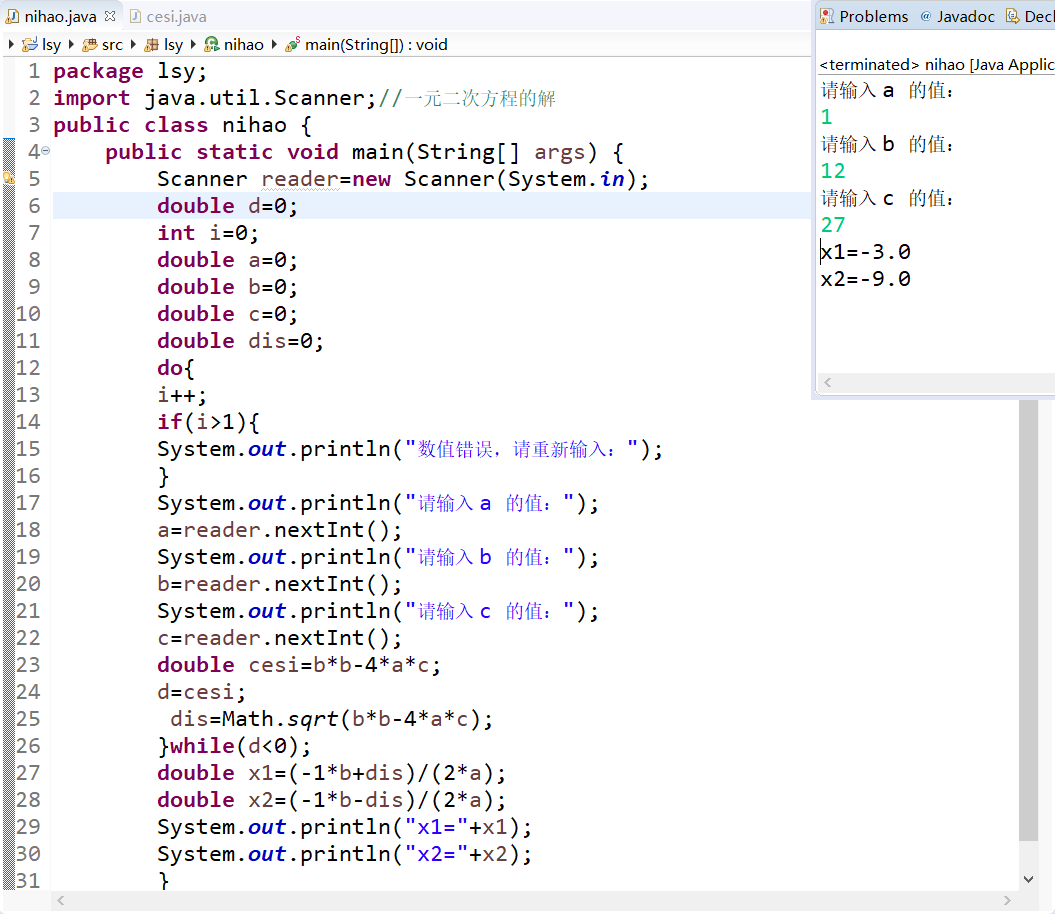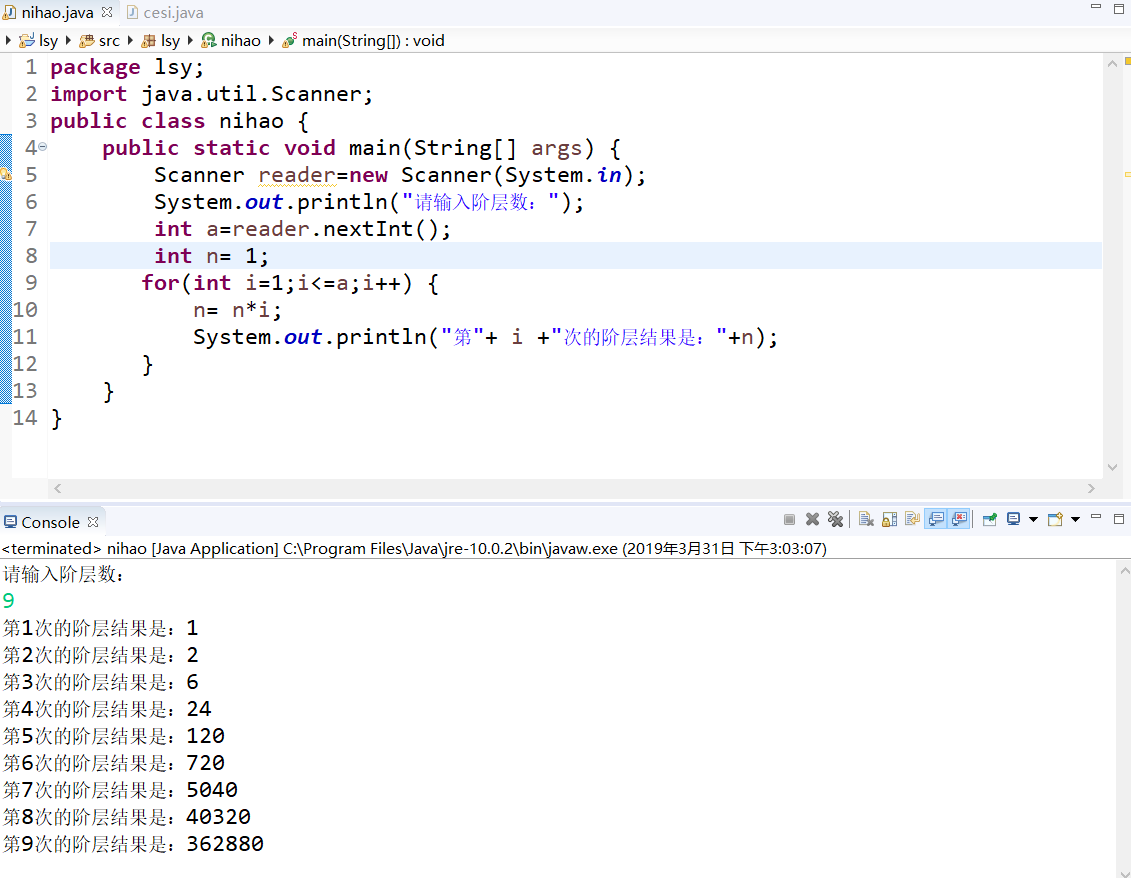• java解一元二次方程，swing界面，输入a,b,c三个值解方程
• 用求根公式法解一元二次方程的一般步骤为： ①把方程化成一般形式 ，确定a，b，c的值（注意符号）； ②求出判别式 的值，判断根的情况； ③在 （注：此处△读“德尔塔”）的前提下，把a、b、c
什么是一元二次方程 ：（来自百度百科）
只含有一个未知数（一元），并且未知数项的最高次数是2（二次）的整式方程叫做一元二次方程。标准形式为：ax²+bx+c=0（a≠0）。

用求根公式法解一元二次方程的一般步骤为：
①把方程化成一般形式，确定a，b，c的值（注意符号）；
②求出判别式的值，判断根的情况；
③在（注：此处△读“德尔塔”）的前提下，把a、b、c的值代入公式进行计算，求出方程的根。
判别式：
一元二次方程的根与根的判别式
有如下关系：①当时，方程有两个不相等的实数根；
②当时，方程有两个相等的实数根；
③当时，方程无实数根，但有2个共轭复根。
#include <stdio.h>
#include <math.h>

int main()
{
int a,b,c;
float x1,x2,d;
printf("输入方程的三个系数:");
scanf("%d %d %d",&a,&b,&c);
printf("方程：");
printf("%dx^2",a);
if(b>0) printf("+");
printf("%dx",b);
if(c>0) printf("+");
printf("%d的解为： ",c);

if(a!=0)
{
d=sqrt(b*b-4*a*c);
if(d>0)//判别式大于零，有两个不相等的实数根
{
x1=(-b+d)/(2*a);
x2=(-b-d)/(2*a);
if(x1<x2)
printf("x1=%0.2f x2=%0.2f\n",x2,x1);
else
printf("x1=%0.2f x2=%0.2f\n",x1,x2);
}
else if(d==0)//判别式等于零，有两个相等的实数根
{
x1=(-b+d)/(2*a);
printf("x1=x2=%0.2f",x1);
}
else//判别式小于零，在实数范围内无解，有两个共轭复根
printf("方程在实数范围内无解\n");

}
else
printf("不是一元二次方程\n");
return 0;
}
测试1：输入方程的三个系数:1 -5 6
方程：1x^2-5x+6的解为： x1=3.00 x2=2.00测试2：
输入方程的三个系数:1 2 1
方程：1x^2+2x+1的解为： x1=x2=-1.00测试3：
输入方程的三个系数:1 2 3
方程：1x^2+2x+3的解为： 方程在实数范围内无解
java实现：
package Ctojava;

import java.util.Scanner;

{
public static void main(String[] args)
{
System.out.print("输入一元二次方程组的系数：");
int a=0,b=0,c=0;
Scanner scanner=new Scanner(System.in);
a=scanner.nextInt();
b=scanner.nextInt();
c=scanner.nextInt();
int k=b*b-4*a*c;
System.out.println("K=b*b-4*a*c="+k);
double x1;
double x2;
System.out.print("方程"+a+"x^2");
if(b>0)
System.out.print("+");
System.out.print(b+"x");
if(c>0)
System.out.print("+");
System.out.println(c+"的解为：");

if(k>0)//有两个根
{
x1=(-b+Math.sqrt(k))/2*a;
System.out.print("x1="+x1);
x2=(-b-Math.sqrt(k))/2*a;
System.out.print(", x2="+x2);
}
else if(k==0)
{
x1=(-b+Math.sqrt(k))/2*a;
System.out.println("x1=x2="+x1);
}
else
{
System.out.print("在实数范围内无解");
}
}
}
测试1：
输入一元二次方程组的系数：1 2 4
K=b*b-4*a*c=-12
方程1x^2+2x+4的解为：
在实数范围内无解测试2：
输入一元二次方程组的系数：1 5 6
K=b*b-4*a*c=1
方程1x^2+5x+6的解为：
x1=-2.0, x2=-3.0测试3：
输入一元二次方程组的系数：1 2 1
K=b*b-4*a*c=0
方程1x^2+2x+1的解为：
x1=x2=-1.0



展开全文• 该楼层疑似违规已被系统折叠隐藏此楼查看此楼publicclassTest2{//如果要求...自己可以加上publicstaticvoidmain(String[]args){System.out.println("---求二次方程式的根---");System.out.println("请输入a*x*　ｘ　...
该楼层疑似违规已被系统折叠 隐藏此楼查看此楼public class Test2 {//如果要求条件多，那就自己加上就行了。这里不过a == 0或者b ==0情况。自己可以加上public static void main(String[] args) {System.out.println("---求二次方程式的根---");System.out.println(" 请输入 a * x *　ｘ　＋　ｂ * x + c = 0 的a ：");Scanner scanner = new Scanner(System.in);double a = scanner.nextDouble();System.out.println(" 请输入 a * x *　ｘ　＋　ｂ * x + c = 0 的b ：");double b = scanner.nextDouble();System.out.println(" 请输入 a * x *　ｘ　＋　ｂ * x + c = 0 的c ：");double c = scanner.nextDouble();double f = b * b - 4 * a * c;if (f System.out.println("此方程没有根");} else if (f == 0) {double result = (-b+Math.sqrt(b * b - 4 * a * c)) / 2*a;System.out.print("此方程有俩个相等的根，他们是：x1 = x2 = " + result);} else if (f > 0) {double result1 = (-b+Math.sqrt(b * b - 4 * a * c)) / 2*a;double result2 = (-b-Math.sqrt(b * b - 4 * a * c)) / 2*a;System.out.println("此方程有俩个不相等的根分别是：result1 = " + result1 + "result2 = " + result2);}}}
展开全文• public class Helloword { ... System.out.println("请输入二次幂参数："); int a =scan.nextInt(); System.out.println("请输入一次幂参数:"); int b =scan.nextInt(); System.out.println("请输入c：")
public class Helloword {
public static void main(String[] args) {
Scanner scan =new Scanner(System.in);
System.out.println("请输入二次幂参数：");
int a =scan.nextInt();
System.out.println("请输入一次幂参数:");
int b =scan.nextInt();
System.out.println("请输入c：");
int c =scan.nextInt();
System.out.println("方程为："+a+"x^2+"+b+"x+"+c+"=0");
if(a==0&&b!=0){
int x;
x=-(b/c);
System.out.println("方程解为："+x);
}else{
int d = (b*b-(4*a*c));
int f =(int)Math.sqrt(d);
if(d>0)
System.out.println("方程解为："+(-b+f)/(2*a)+"和"+(-b-f)/(2*a));
else if(d<0)
System.out.println("方程解无解：");
else
System.out.println("方程解为："+(-(b/(2*a))));
}
}

}


展开全文• 1.编程序，输入 a,b,c，求方程 ax2+bx+c=0 的解。 源码: package lsy;...//一元二次方程的解 public class nihao { public static void main(String[] args) { Scanner reader=new Scanner(System....
1.编程序，输入 a,b,c，求方程 ax2 +bx+c=0 的解。源码:

package lsy;
import java.util.Scanner;//一元二次方程的解
public class nihao {
public static void main(String[] args) {
double d=0;
int i=0;
double a=0;
double b=0;
double c=0;
double dis=0;
do{
i++;
if(i>1){
System.out.println("数值错误，请重新输入：");
}
System.out.println("请输入 a 的值：");
System.out.println("请输入 b 的值：");
System.out.println("请输入 c 的值：");
double cesi=b*b-4*a*c;
d=cesi;
dis=Math.sqrt(b*b-4*a*c);
}while(d<0);
double x1=(-1*b+dis)/(2*a);
double x2=(-1*b-dis)/(2*a);
System.out.println("x1="+x1);
System.out.println("x2="+x2);
}
}

2.阶层源码：

package lsy;
import java.util.Scanner;
public class nihao {
public static void main(String[] args) {
System.out.println("请输入阶层数：");
int n= 1;
for(int i=1;i<=a;i++) {
n= n*i;
System.out.println("第"+ i +"次的阶层结果是："+n);
}
}
}

展开全文• package net.xmh.lesson3; public class Fc{ int a,b,c; double gen() {double i; double j=1.0; double k=1.0; i=bb-4ac; if(i>=0) j=((-b)+Math.sqrt(i))/2a; k=((-b)-Math.sqrt(i))/2*a;...public...
• 方程两个虚根为：NaNi，NaNi这是怎么回事？？？(编译没问题 运行出上面的语句)classEquation{privatefloata,b,c;staticdoublez,p;publicEquation(){}publicEquation(floata,floatb,floatc){this.a=a;this.b=b;this...
• * @desc 一元三次方程，二次方程，和一次方程求解工具类 */ public class EquationCalculation { /* 计算一元三次方程最大实根 * 一元三次方程(ax3+bx2+cx+d＝0)的盛金公式解题法 * 输入：参数a,b,c,d * 1)：...算法
• 给定一个字符串，代表一个一元一次方程。如果有解求解，输出格式“x=数字” ，如果解的个数无穷，输出 “infinite solutions”。如果没有解输出“no solution” 字符串长度不超过 256 。 输入格式： 输入一个一元...
• 8.解一元二次方程。 输入一元二次方程的a,b,c三个系数，解一元二次方程 ax^2+bx+c=0,输出两个根 package study; import java.util.Scanner; public class demo1 { public static void main(String[] args...
• 两个解一个解无解 import java.util.Scanner; public class thirdegmath { public static void main(String[] args) { Scanner input=new Scanner(System.in); System.out.print("enter a,b,c:"); int...
• 所以今天我用Java语言实现了当年的解一元二次方程的程序，思想还是以前的思想，只不过是语法不一样罢了，换汤不换药。 思想 授人以鱼不如授人以渔。首先先说一下写这个程序的需求和思想。 需求：当我们遇到
• 代数：解一元二次方程）可以使用下面的公式求一元二次方程 ax2+bx+c=0 的两个根： b2-4ac 称作一元二次方程的判别式。 如果它是正值，那么一元二次方程就有两个实数根。 如果它为 0， 方程式就只有一个根。如果它是...
• 那时候通过看视频和《C primer plus》写了一个解一元二次方程的程序，从此走上了吊打大学同班同学的路……但是那次是用C语言写的，如今白云苍狗，我已经不是曾经的那个我了，但我还是一如既往的废物……Java已是我...
• 通过java解一元二次方程，考虑（a、b、c的各种情况）
• import java.util.Scanner; public class helloworld{ public static void main(String[] args) { System.out.print("Enter a,b,c: " ); Scanner input = new Scanner(System.in); try { double...
• 你只需要输入a,b,c三个系数,就可以求出这条一元二次方程的解
• System.out.println("方程有两个解："+ (-b+Math.sqrt(d))/(2*a) +"和"+ (-b-Math.sqrt(d))/(2*a)); } //Math.sqrt()用来开平方 方程要化成一般式ax^2+bx+c=0 方程结果是小数表示 ，还有待改进
• 解一元次方程（一）——合并同类项》是人教版七年级上册第三章第节的内容，本节课的内容主要是会利用合并同类项来解一元次方程，是在学生已经学习了一元一次方程的概念，等式的性质及会合并同类项等的基础上...
• //调用排序测试函数 } } 运行结果： 2、解一元二次方程 public class equation_test { void equation_test()//解方程的函数 { double x1,x2;//定义两个根 System.out.println("求方程3x^2+4x-1=0的根");//...# java解一元二次方程java 订阅